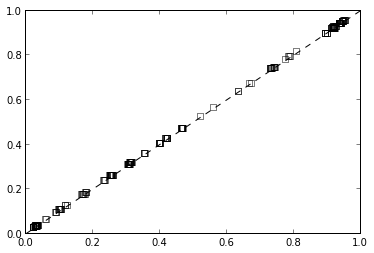In :
!date

Sat Apr 13 13:33:11 PDT 2013

From: [email protected] [mailto:[email protected]] On Behalf Of Tommy Engström
Sent: Wednesday, April 10, 2013 2:40 PM
To: [email protected]
Subject: [pymc] Naive bayes in PyMC

I'm completely new to PyMC so this is most likely a trivial question but I haven't managed to figure it out.

I thought I'd start my pymc journey by building a naive bayes classifier using pymc but I'm having trouble understanding how to do that.

The problem:

1000 observations of 10 attributes + 1 target attribute, all booleans.
How can fit the network once I have it? I need to be able to set all 11 attributes at once. Or am I just thinking completely wrong here?

Thankful for any guiding words
In :
import pymc as mc


Naive Bayes in its simplest form is a prediction model for categorial data.

I happen to have code from a recent project which simulates data of this form from an interesting distribution:

In :
import random
def simulate(n, p, seed):
random.seed(123456+seed)
mc.np.random.seed(123456+seed)

# make A clusters, beta distributed
A = 5
X_true = mc.rbeta(.5, .5, size=(A,p))
y_true = mc.rbeta(1, 1, size=A)

X = zeros((n,p))
p_true = zeros(n)
for i in range(n):
a_i = random.randrange(A)
X[i] = mc.rbernoulli(X_true[a_i])
p_true[i] = y_true[a_i]

y = mc.rbinomial(1, p_true)

test = random.sample(range(n), n/4)
train = list(set(range(n)) - set(test))

X_train = X[train]
y_train = y[train]

X_test = X[test]
y_test = y[test]

return locals()

In :
n = 1000
p = 10
data = simulate(n=n, p=p, seed=0)


As mentioned on the PyMC list, it is not necessary to use PyMC for naive bayes prediction, and scikits-learn has a very simple way to handle this:

In :
import sklearn.naive_bayes

In :
nb = sklearn.naive_bayes.BernoulliNB(alpha=0, fit_prior=False)
nb.fit(data['X_train'], data['y_train'])

Out:
BernoulliNB(alpha=0, binarize=0.0, class_prior=None, fit_prior=False)
In :
y_pred = nb.predict_proba(data['X_test'])

In :
# accuracy
mean((y_pred[:,1] >= .5) == data['y_test'])

Out:
0.66000000000000003

But there is nothing wrong with implementing the naive bayes model in PyMC; it could be a good project for getting started.

Formulating the naive bayes predictor in PyMC requires writing this machine learning standard in the language of Bayesian statistics, specifically, the "data likelihood" of $X_j | y$: $$X_j \sim Be(\alpha_j y + \beta_j (1-y)),$$ where $\alpha_j$ is the probability of $X_j$ being 1 when $y$ is 1, and $\beta_j$ is the probability of $X_j$ being 1 when $y$ is 0.

The tradition in ML is not to think much about the prior distribution of $y$, and so I won't: $$y_i \sim Be(.5).$$

In :
n_test = len(data['X_test'])
y = [mc.Bernoulli('y_%d'%i, .5) for i in range(n_test)]

alpha = empty(p)
beta = empty(p)
for j in range(p):
# alpha[j] is Pr[X_j = 1 | y = 1] in training data
alpha[j] = (data['X_train'][:,j] * data['y_train']).sum() / data['y_train'].sum()

# beta[j] is Pr[X_j = 1 | y = 0] in training data
beta[j] = (data['X_train'][:,j] * (1-data['y_train'])).sum() / (1-data['y_train']).sum()

X = [mc.Bernoulli('X_%d_%d'%(i,j), alpha[j]*y[i]+beta[j]*(1-y[i]), value=data['X_test'][i,j], observed=True) for i in range(n_test) for j in range(p)]

In :
mcmc = mc.MCMC([y, X])
%time mcmc.sample(10000)

[****************100%******************]  10000 of 10000 complete CPU times: user 19min 19s, sys: 168 ms, total: 19min 19s
Wall time: 19min 23s

In :
plot([y_i.stats()['mean'] for y_i in y], y_pred[:,1], 's', mec='k', color='none')
plot([0,1], [0,1], 'k--')

Out:
[<matplotlib.lines.Line2D at 0x1b076a10>]In :